Refer to our Texas Go Math Grade 4 Answer Key Pdf to score good marks in the exams. Test yourself by practicing the problems from Texas Go Math Grade 4 Unit 3 Assessment Answer Key.

Vocabulary

• perimeter
• area
• formula
• pattern
• square unit (sq un)

Choose the best term from the box.

Question 1.
A set of symbols that expresses a mathematical rule is called a _____________ .
Formula
Explanation:
A set of symbols that expresses a mathematical rule or principle;
for example, the formula for the area of a rectangle is a = l x w,
where a is the area, l the length, and w the width.

Question 2.
The ____________ is the distance around a figure.
Perimeter
Explanation:
The perimeter of a two-dimensional figure is the distance covered around it.
It defines the length of shape, whether it is a triangle, square, rectangle or a circle.

Question 3.
A ___________ is an ordered set of numbers or objects.
Pattern
Explanation:
Math patterns are sequences that repeat based on a rule, and a rule is a set way to calculate or solve a problem.

Concepts and Skills

Use the rule to write the first ten terms in the pattern. Describe another
pattern in the numbers.

Question 4.
Rule: Add 8. First term: 7Explanation:
Rule: Add 8. First term: 7
t = 7,8,9,10
Input = t + 8
Output = 7 + 8 = 15
Input = t + 8
Output = 8 + 8 = 16
Input = t + 8
Output = 9 + 8 = 17
Input = t + 8
Output = 10 + 8 = 18

Question 5.
Find a rule. Use your rule to write an expression.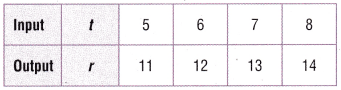Rule: Add 6. First term: 5
Explanation:
Rule: Add 6. First term: 5
t = 5,6,7,8,
Input = t + 6
Output = 5 + 6 = 11
Input = t + 6
Output = 6 + 6 = 12
Input = t + 6
Output = 7 + 6 = 13
Input = t + 6
Output = 8 + 6 = 14

Question 6.
Use the rule to complete the input/output table.
Rule: The output is a × 5.b = a x 5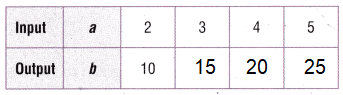Explanation:
Rule: b = a x 5
a = 2,3,4,5
Input = a x 5
Output = 2 x 5 = 10
Output = 3 x 5 = 15
Output = 4 x 5 = 20
Output = 5 x 5 = 25

Find the perimeter and area of the rectangle or square.

Question 7.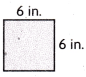Perimeter = 24 inches
Area = 36 inches
Explanation:
Perimeter of a Square = 4 × side
= 4 x 6 = 24
Area of a Square = side × side
= 6 x 6 = 36

Question 8.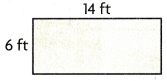Perimeter = 40 feet
Area = 84 feet
Explanation:
Area of a rectangle = l × w
= 6 x 14 = 84

Perimeter of a rectangle = 2 × ( l + w )
= 2 x (6 + 14)
= 2 x 20 = 40

Question 9.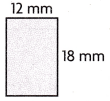Perimeter = 60 mm
Area = 216 mm
Explanation:
Area of a rectangle = l × w
= 18 x 12 = 216

Perimeter of a rectangle = 2 × ( l + w )
= 2 x (18+ 12)
= 2 x 30 = 60

Question 10.
Melissa wants to use a formula to find the perimeter of the figure below. Which formula should Melissa use?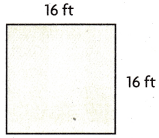(A) P = 2 + w + 2 + l
(B) P = l + w
(C) P = l × w + l × w
(D) P = 4s
Option(D)
Explanation:
The picture above is square, as all sides are equal.
so, the perimeter of square is 4s.

Question 11.
Martin buys four packs of baseball cards. Each pack has 12 cards in it. He then buys 3 more packs of cards with 8 cards in each pack. How many baseball cards did Martin buy? Use strip diagrams or equations to help you solve.
(A) 96
(B) 72
(C) 27
(D) 66
Option(B)
Explanation:
Martin buys four packs of baseball cards.
Each pack has 12 cards in it.
He then buys 3 more packs of cards with 8 cards in each pack.
Number of baseball cards Martin buy
(4 x 12) + (3 x 8) = n
48 + 24 = n
72 = n

Question 12.
Greg drew the sketch of his garden below. What is the area of Greg’s garden?(A) 36 square feet
(B) 200 square feet
(C) 288 square feet
(D) 72 square feet
Option(C)
Explanation:
Greg’s garden is in rectangle shape.
Area of rectangle = l x w
length = 12; width = 24
l x b = 12 x 24 = 288 square feet.

Question 13.
Pablo is using this strip diagram to solve a problem. Which equation is shown by the strip diagram?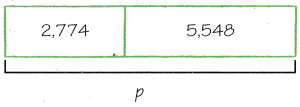(A) 5,548 – 2,774 = p
(B) 5,548 – p = 2,774
(C) 2,774 + 5,548 = p
(D) 2,774 + p = 5,548
option(C)
Explanation:
Algebraic equation p = (a + b)
2,774 + 5,548 = p

Question 14.
Marcel had 72 stickers. He gave 14 of his stickers to his sister. Then he gave 27 stickers to his brother. How many stickers does Marcel have left? Use strip diagrams or equations to help you solve.
(A) 58
(B) 31
(C) 45
(D) 113
Option(B)
Explanation:
He gave 14 of his stickers to his sister.
Then he gave 27 stickers to his brother.
Number of stickers left with Marcel
72 – (14 + 27) = n
72 – 41 = n
31 = n

Question 15.
Gabby wants to put a fence around the perimeter of her backyard. She drew a sketch of her backyard to determine the perimeter. What is the perimeter of Gabby’s backyard?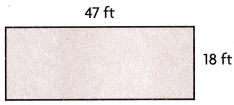(A) 130 ft
(B) 611 ft
(C) 112 ft
(D) 83 ft
Option(A)
Explanation:
Gabby’s backyard is in the shape of rectangle.
Perimeter of rectangle = 2 (l + w)
length = 18 ft; width = 47 ft
p = 2x (18 + 47)
= 2 x 65
= 130 ft

Question 16.
Dan wants to find the area of his rectangular room so he will know how much carpet to buy. Which formula should he use to find the area of his room?
(A) A = l + w
(B) A = l × w
(C) A = (l × w) + (l × w)
(D) A = (2 × w) + (2 × l)
Option(B)
Explanation:
Area of rectangle = l x w
l = length; w = width

Question 17.
Erica has 144 lollipops. She wants to put the lollipops into 8 different bags, with each bag having the same number of lollipops. Once Erica has all the lollipops in the 8 bags, she takes 2 lollipops out of each bag. How many lollipops are in each of the 8 bags? Use strip diagrams or equations to help you solve.
(A) 12
(B) 16
(C) 20
(D) 18
Option(B)
Explanation:
Erica has 144 lollipops.
She wants to put the lollipops into 8 different bags, with each bag having the same number of lollipops.
Once Erica has all the lollipops in the 8 bags, she takes 2 lollipops out of each bag.
Number of lollipops in each of the 8 bags
(144 ÷ 8) – 2= n
18 – 2 = n
16 = n

Question 18.
Karen walks 4 miles on Monday. For the next 7 days, she walks 2 miles each day. How many miles total does Karen walk during the 8 days?
(A) 18 miles
(B) 14 miles
(C) 34 miles
(D) Not here
Option(A)
Explanation:
Karen walks 4 miles on Monday.
For the next 7 days, she walks 2 miles each day.
Total miles Karen walk during the 8 days
4 + (7 x 2) = n
4 + 14 = n
n = 18 miles

Question 19.
During recess, Gretchen walked around the perimeter of the playground. When she got home, she drew a sketch of the playground to help her determine how far she walked during recess. What is the perimeter of the playground?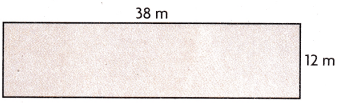(A) 50 m
(B) 88 m
(C) 62 m
(D) 100 m
Option(D)
Explanation:
Gretchen playground is in the shape of rectangle.
Perimeter of rectangle = 2 (l + w)
length = 12 m ; width = 38 m
p = 2x (12 + 38)
= 2 x 50
= 100 m

Question 20.
Van is buying new tires for some cars at his store. The input-output table below shows the number of cars, c, and the number of tires, t, on each car. Using the rule c × 4, how many tires does Van have to buy to replace all the tires on 6 cars?(A) 12
(B) 16
(C) 24
(D) 20
Option (C)
t = c x 4
Explanation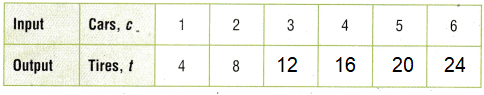Question 21.
An art museum adds 3 new pieces of art each month. If the museum starts with 75 pieces and the pattern continues, write the numbers in the pattern for the next 8 months. Describe another pattern in the numbers.Use the rule to complete the input/output table.
Rule: The output is a + 3.
Explanation:
Rule: Add a. First term: 75
a = 75,78,81,84,87,90,93,96,99
Input = a+ 3
Output = b + 3
Output = 75 + 3 = 78
Output = 78 + 3 = 81
Output = 81 + 3 = 84
Output = 84+ 3 = 87
Output = 87 + 3 = 90
Output = 90 + 3 = 93
Output = 93 + 3 = 96
Output = 96 + 3 = 99

b = a + 3

Scroll to Top